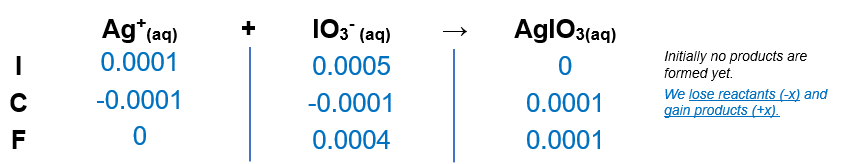# Problem: A 50.0-mL sample of 0.00200 M AgNO3 is added to 50.0 mL of 0.0100 M NaIO 3. What is the equilibrium concentration of Ag+ in solution? (Ksp for AgIO3 is 3.0 X 10-8.)

###### FREE Expert Solution

(1)Calculate moles of Ag+ and IO3-

mol Ag+ = 0.0001 mol

mol IO3-= 0.0005 mol

(2) Construct an ICF chartAt this point, no Ag+ left

97% (57 ratings)###### Problem Details

A 50.0-mL sample of 0.00200 M AgNO3 is added to 50.0 mL of 0.0100 M NaIO 3. What is the equilibrium concentration of Ag+ in solution? (Ksp for AgIO3 is 3.0 X 10-8.)